# Infosys Pseudocode Previous Year Questions

18 January 2023

Infosys Pseudocode Previous Year Questions

Infosys Pseudocode  Questions

Q1) What will be the output of the following pseudocode for p = 3 and q = 4?

int fun1(int p, int q)

if(q EQUALS 0)

return 0

if(q mod 2 EQUALS 0)

return fun1(p + p, q/2) .

return fun1(p + p, q/2) + p

End function fun1()

A. None of the options

B. 7

C. 12

D. 8

Explanation:

Here initially p =3, q=4. In this question we are using recursive function.

If ( 4 == 0 ) is false so it will go to next if condition

If ( 4 % 2 == 0 ) -> if(true) so, here it will return fun1(p + p, q/2 )

That is fun1(6,2) now the new value of p=6 and q=2

Again same if condition will work as earlier

If(2 % 2 == 0) ->of (true) so here it will return fun1(6 + 6 , 2/2)

That is fun1(12,1) again p=1, q=1.

This time both if condition will return false so it will go to the last return statement.

That is

Return fun1(p+p, q/2) + p

Fun1(12+12, 1/2) + 12 ->fun(24,0) + 12

This time q is 0. So, if(q==0) will return 0. That means fun1(24,0) this function will return 0

So finally 0 + 12 = 12

Q2) What will be the output of the following pseudocode?

Integer x, y, Z, a

Set x=2,y=1,z=5

a = (x AND y) OR (z + 1)

Print a

A. 5

B. 3

C. 2

D. 1

Explanation:

Here x = 2, y = 1, z = 5

a = (2 && 1) || (5 + 1)

= (1) || (6)

= 1

So it will print 1

Q3) What will be the output of the following pseudocode for a = 2, b = 3?

doSomething(Integer a, Integer b)

if(b EQUALS 1)

return 0

else

return a+ doSomething(a, b-1)

End function doSomething()

A. 3

B. 4

C. 2

D. 1

Explanation:

Here a = 2, b = 3. We are using recursive function here.

If(3==1) ->false

So it will go to else part

return 2 + doSomething(2, 3-1)

doSomething(2, 2) -> return 2 + doSomething(2,2-1)

doSomething(2, 1) -> if(1==1) is true now so it will return 0.

Now the function will return the values

doSomething(2, 1) -> returns 0

doSomething(2, 2) -> returns 2

doSomething(2, 3) -> returns 4

So by the end of the function it will return 4

Q4) What will be the output of the following pseudocode?

Integer a, n, sum, q, r

Set a=123, n=0, sum=0

Set q=a

while (q mod 10 NOT EQUALS 0)

n=n+1

q=q/10

End while

Print n

while (a greater than 0)

r=a mod 10

sum =sum + r

a =a/power(10, n- 1)

End while

Print sum

A. 3 2

B. 6 4

C. 6 5

D. 3 4

Explanation:

Here a= 123, n=0, sum=0, q=123

while( 123 % 10 != 0 ) -> n = 0+1 =1 , q = 123/10 = 12

while( 12 % 10 !=0 ) -> n = 1 + 1 = 2 , q = 12/10 = 1

while (1% 10 != 0 ) -> n = 2 +1 = 3, q = 1/10 = 0

Next time while loop will become false

Print n will print 3

while(123>0) -> r = 123%10 = 3, sum = 0+3 = 3, a = 123/100 = 1

while(1>0) -> r = 1%10 = 1 , sum = 3 + 1 = 4, a = 1 / 100 = 0

next time while loop will be false

print sum will print 4

Q5) What will be the output for the following pseudocode?

Integer a=5,b=8,c

if(a>8 OR b < 7)

c=a+b

elseif(a + b > 9)

c=a-b

else

a=a+5

c=c-2

end if

a=a+b

Print a and c

b=b-5

[Note: OR — The logical Or operator returns the Boolean value if either or both operands are true and returns false otherwise.]

A. None of the mentioned

B. 5 -3

C. 5 1

D. 13 -3

Explanation:

Here a = 5, b=8

If(5>8 || 8<7) -> false

Else if(5+8 > 9) -> true -> c = 5-8 = -3

After the end of if condition

a = a+b à-> 5 + 8 =13

print a, c will print 13 and -3

Q6) What will happen when following pseudocode is executed?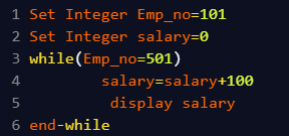A. Code executes successfully and value of salary is displayed once.

B. Code executes successfully and nothing is displayed.

C. Code executes successfully and value of salary is displayed infinite number of times.

D. Compile time error.

Explanation:

Inside the while loop we are using the assignment operator so the loop is going to work for infinite times and all the time salary will be printed by adding 100 to it.

Q7) What will happen when following program is executed?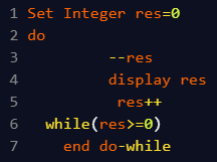A. Code will run infinite number of times.

B. The program will not enter the loop.

C. Code will execute and value of res will be displayed twice.

D. Code will execute and value of res will be displayed once.

Explanation:

Here inside the do while loop the value of res is getting decremented and incremented alternatively, so, always the while condition will be true. And thus the loop is going to work for infinite times.

Q8) How many times will ‘#’ be displayed?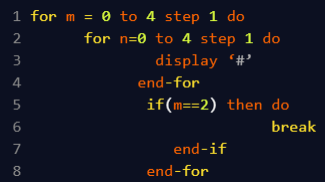A. 15

B. 1

C. 2

D. 8

Explanation:

The outer and inner for loop is varying from 0 to 4 so, totally five times each loop will work

Keeping m=0 , n loop will work 5 times so # will be printed 5 times

Then again keeping m =1, n loop will work 5 times so again # will be printed for 5 times.

Now keeping m =2, n will work for 5 times, again # will be printed for 5 times.

But this time if condition will be true and it will break the outer loop.

So totally 15 times # will be printed.

Q9) What will be the output of the following pseudocode?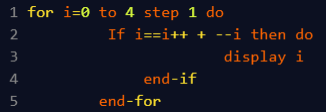A. 0

B. 3

C. 1

D. 2

Explanation:

Here only when i value is 0 the if condition will be true. All the values of i the if condition will result in false. So, output is 0

Q10) What will be the output of the following pseudocode?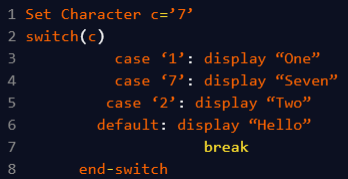A. SevenTwoHello

B. OneHello

C. SevenTwo

D. Seven

Explanation:

Here c is 7 so, switch (7) will go to the case with 7 as the label.

Following to case 7 , 2 and default case all values will be printed as there is no break statement in between.

Q11) What will be the output of the following pseudocode?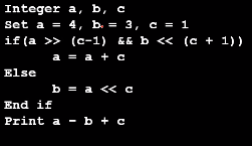A. 3

B. 5

C. 8

D. None of these

Explanation:

Here a= 4, b = 3, c=1.

If(4>>(1-1) && (3<<(1+1)) -> if( 4 && 12) à true

So, a= 4+1 = 5

Next it will print a-b+c -> 5-3+1 = 3

Q12) What will be the output of the following pseudocode?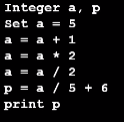A. 0

B. 1

C. 2

D. 7

Explanation:

Here initially a = 5

a = 5+1 =6

a = 6*2 = 12

a = 12/2 = 6

p = 6/5 + 6 -> p = 1 + 6 =7

Q13) What will be the output of the following pseudocode?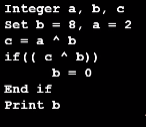A. 3

B. 0

C. 10

D. 2

Explanation:

Here b = 8, a = 2

c = a ^ b -> 2 ^ 8 = 10

if(10^8) -> if(2) -> true so, b=0

Q14) What will be the output of the following pseudocode?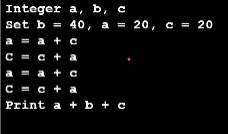A. 40

B. 100

C. 300

D. None of these

Explanation:

Here b=40, a=20, c=20

a = 20 + 20 = 40

c = 20 + 40 =60

a = 40 + 60 = 100

c = 60 + 100 = 160

print a + b + c -> 100 + 40 + 160 = 300

Q5) What will be the output of the following pseudocode?A.1

B. 2

C. 0

D. None of these

Explanation:

Here a= 1, b=1

a = (1^1) & (1) + (1^1) & (1)

= 0 & 1 + 0 & 1

= 0

Print 0 + 1 = 1

##